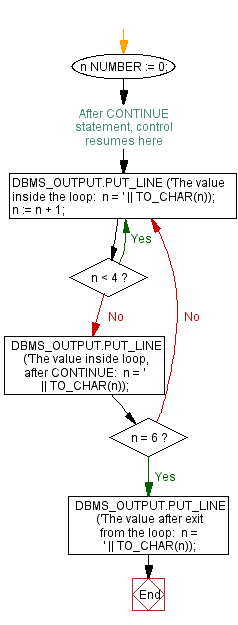﻿ PL/SQL Control Statement: Using loop with CONTINUE WHEN statement - w3resource# PL/SQL Control Statement Exercises: Using loop with CONTINUE WHEN statement

## PL/SQL Control Statement: Exercise-22 with Solution

Write a program in PL/SQL using loop with CONTINUE WHEN statement.

Sample Solution:

PL/SQL Code:

``````DECLARE
n NUMBER := 0;
BEGIN
LOOP -- After CONTINUE statement, control resumes here
DBMS_OUTPUT.PUT_LINE ('The value inside the loop:  n = ' || TO_CHAR(n));
n := n + 1;
CONTINUE WHEN n < 4;
DBMS_OUTPUT.PUT_LINE
('The value inside loop, after CONTINUE:  n = ' || TO_CHAR(n));
EXIT WHEN n = 6;
END LOOP;
DBMS_OUTPUT.PUT_LINE ('The value after exit from the loop:  n = ' || TO_CHAR(n));
END;
/
```
```

Flowchart:Sample Output:

```The value inside the loop:  n = 0
The value inside the loop:  n = 1
The value inside the loop:  n = 2
The value inside the loop:  n = 3
The value inside loop, after CONTINUE:  n = 4
The value inside the loop:  n = 4
The value inside loop, after CONTINUE:  n = 5
The value inside the loop:  n = 5
The value inside loop, after CONTINUE:  n = 6
The value after exit from the loop:  n = 6

PL/SQL procedure successfully completed.```

Improve this sample solution and post your code through Disqus

What is the difficulty level of this exercise?

﻿